# Class 8 maths chapter 2 exercise 2.3 solutions

In this page we have class 8 maths chapter 2 exercise 2.3 solutions .This exercise has questions about simple linear equation where we have linear expression on Present on both the left and right side of the equation. Hope you like them and do not forget to like , social share and comment at the end of the page.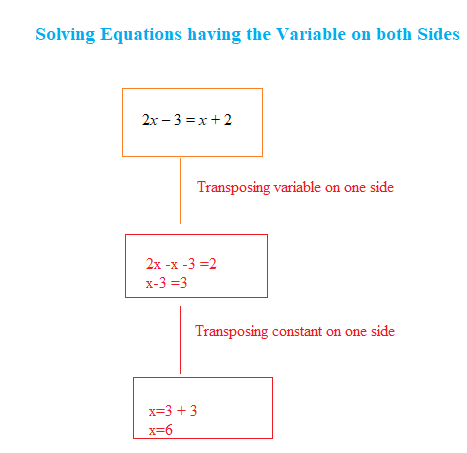Tips for this NCERT Exercise
(1) Validation or check result means to verify the answer by putting the values in the linear equation
(2) Calculate the LHS(Left Hand side) by substituting the variable and similary calculate the RHS(Right hand side) by substituting the variable
(3) Both the LHS and RHS should be equal

## Class 8 maths chapter 2 exercise 2.3 solutions

Question 1
Solve and check result: $3x= 2x+ 18$
$3x= 2x+ 18$
Transposing 2x to L.H.S, we obtain
$3x- 2x= 18$
x= 18
Let us evaluate both the LHS and RHS for validate the answer
L.H.S = 3x= 3 × 18 = 54
R.H.S = 2x+ 18 = 2 × 18 + 18 = 36 + 18 = 54
L.H.S. = R.H.S.
It proves that result is correct

Question 2
Solve and check result: $5t- 3 = 3t- 5$
$5t- 3 = 3t- 5$
Transposing 3t to L.H.S and -3 to R.H.S, we obtain
$5t- 3t= -5 - (-3)$
2t= -2
Dividing both sides by 2
t= -1
Let us evaluate both the LHS and RHS for validate the answer
L.H.S = 5t- 3 = 5 × (-1) - 3 = -8
R.H.S = 3t- 5 = 3 × (-1) - 5 = - 3 - 5 = -8
L.H.S. = R.H.S.
It proves that result is correct

Question 3
Solve and check result
$5x+ 9 = 5 + 3x$
$5x+ 9 = 5 + 3x$
Transposing 3x to L.H.S and 9 to R.H.S, we obtain
$5x- 3x= 5 - 9$
2x= -4
Dividing both sides by 2, we obtain
x= -2
Let us evaluate both the LHS and RHS for validate the answer
L.H.S = 5x+ 9 = 5 × (-2) + 9 = -1
R.H.S = 5 + 3x= 5 + 3 × (-2) = -1
L.H.S. = R.H.S.
It proves that result is correct

Question 4
Solve and check result:
$4z+ 3 = 6 + 2z$
$4z+ 3 = 6 + 2z$
Transposing 2z to L.H.S and 3 to R.H.S, we obtain
$4z- 2z= 6 - 3$
2z= 3
Dividing both sides by 2, we obtain
z=3/2
Let us evaluate both the LHS and RHS for validate the answer
L.H.S = 4z+ 3 = 4 × (3/2)+ 3 = 6 + 3 = 9
R.H.S = 6 + 2z= 6 + 2 × (3/2)= 6 + 3 = 9
L.H.S. = R.H.S.
It proves that result is correct

Question 5
Solve and check result:
$2x- 1 = 14 -x$
$2x- 1 = 14 -x$
Transposing x to L.H.S and 1 to R.H.S, we obtain
$2x+x= 14 + 1$
3x= 15
Dividing both sides by 3, we obtain
x= 5
Let us evaluate both the LHS and RHS for validate the answer
L.H.S = 2x- 1 = 2 × (5) - 1 = 10 - 1 = 9
R.H.S = 14 -x= 14 - 5 = 9
L.H.S. = R.H.S.
It proves that result is correct

Question 6
Solve and check result:
$8x+ 4 = 3(x- 1) + 7$
$8x+ 4 = 3(x- 1) + 7$
$8x+ 4 = 3x- 3 + 7$
Transposing 3x to L.H.S and 4 to R.H.S, we obtain
$8x- 3x= - 3 + 7 - 4$
$5x= - 7 + 7$
5x=0
x=0
Let us evaluate both the LHS and RHS for validate the answer
L.H.S = 8x+ 4 = 8 × (0) + 4 = 4
R.H.S = 3(x- 1) + 7 = 3 (0 - 1) + 7 = - 3 + 7 = 4
L.H.S. = R.H.S.
It proves that result is correct

Question 7-
Solve and check result: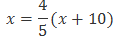Multiplying both sides by 5, we obtain
$5x= 4(x+ 10)$
$5x= 4x+ 40$
Transposing 4x to L.H.S, we obtain
5x- 4x= 40
x= 40
Let us evaluate both the LHS and RHS for validate the answer
L.H.S =x= 40
R.H.S ==40
L.H.S. = R.H.S.
It proves that result is correct

Question 8
Solve and check result: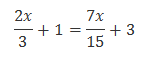Transposing 7x/15 on LHS and 1 on RHS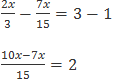Multiplying by 15 on both sides
10x-7x= 30
3x=30
Dividing by 3 on both the sides
x=10
Let us evaluate both the LHS and RHS for validate the answer
L.H.S =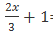=23/3
R.H.S=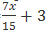=23/3
L.H.S. = R.H.S.
It proves that result is correct

Question 9
Solve and check result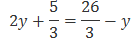Transposingyto L.H.S and5/3 to R.H.S, we obtain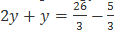3y=21/3
3y=7
Dividing both sides by 3, we obtain
y=7/3
Let us evaluate both the LHS and RHS for validate the answer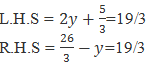L.H.S. = R.H.S.
It proves that result is correct

Question 10
Solve and check result: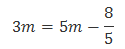Transposing 3m to R.H.S and 8/5 to L.H.S
8/5= 2m
Dividing both sides by 2
m=4/5
Let us evaluate both the LHS and RHS for validate the answer
L.H.S =3m=12/5
R.H.S =5m –(8/5)=12/5
L.H.S. = R.H.S.
It proves that result is correct

## Practice similar type questions

(1) Solve and check the result for $4n +7 = 23$
(2) Solve and check the result $3x - 4 = 1 - 2 x$
(3) Solve and check the result $\frac {m}{2} -11= 2$
(4) Solve and check the result $11y =6y - \frac {1}{3}$
(5) Solve the check the result $\frac {x}{5} + 8=30$

## Summary

1. NCERT Solutions for Linear equations Class 8 maths Chapter 2 Exercise 2.2 has been prepared by Expert with utmost care. If you find any mistake.Please do provide feedback on mail. You can download the solutions as PDF in the below Link also
2. This chapter 2 has total 6 Exercise 2.1,2.2,2.3,2.4,2.5.2.6. This is the Third exercise in the chapter.You can explore previous exercise of this chapter by clicking the link below